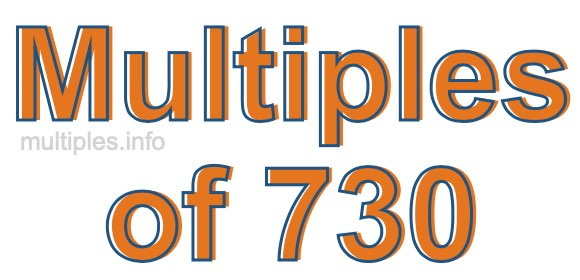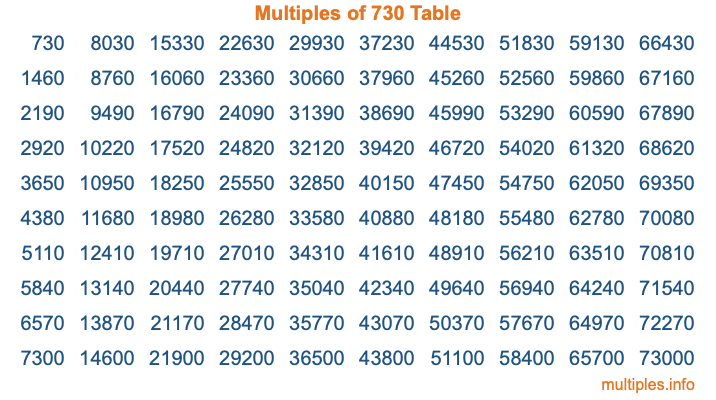Multiples of 730Welcome to the Multiples of 730 page. Here we will first teach you everything you will ever need to know about the multiples of 730, and then give you a study guide summary of everything we taught you to make sure you remember it all. Use this page to look up facts and learn information about the multiples of 730. This page will make you a multiples of seven hundred thirty expert!

Definition of Multiples of 730
Multiples of 730 are all the numbers that when divided by 730 equal an integer. Each of the multiples of 730 are called a multiple. A multiple of 730 is created by multiplying 730 by an integer.

Therefore, to create a list of multiples of 730, you start with 1 multiplied by 730, then 2 multiplied by 730, then 3 multiplied by 730, and so on for as long as you want. Thus, the list of the first five multiples of 730 is 730, 1460, 2190, 2920, and 3650. To see a larger list of multiples of 730, see the printable image of Multiples of 730 further down on this page. We also have a category where you can choose any nth multiple of 730.

Multiples of 730 Checker
The Multiples of 730 Checker below checks to see if any number of your choice is a multiple of 730. In other words, it checks to see if there is any number (integer) that when multiplied by 730 will equal your number. To do that, we divide your number by 730. If the the quotient is an integer, then your number is a multiple of 730.

Is  a multiple of 730?

Least Common Multiple of 730 and ...
A Least Common Multiple (LCM) is the lowest multiple that two or more numbers have in common. This is also called the smallest common multiple or lowest common multiple and is useful to know when you are adding our subtracting fractions. Enter one or more numbers below (730 is already entered) to find the LCM.

Check out our LCM Calculator if you need more details about the Least Common Multiple or if you need the LCM for different numbers for adding and subtraction fractions.

nth Multiple of 730
As we stated above, 730 is the first multiple of 730, 1460 is the second multiple of 730, 2190 is the third multiple of 730, and so on. Enter a number below to find the nth multiple of 730.

th multiple of 730

Multiples of 730 vs Factors of 730
730 is a multiple of 730 and a factor of 730, but that is where the similarities end. All postive multiples of 730 are 730 or greater than 730. All positive factors of 730 are 730 or less than 730.

Below is the beginning list of multiples of 730 and the factors of 730 so you can compare:

Multiples of 730: 730, 1460, 2190, 2920, 3650, etc.

Factors of 730: 1, 2, 5, 10, 73, 146, 365, 730

As you can see, the multiples of 730 are all the numbers that you can divide by 730 to get a whole number. The factors of 730, on the other hand, are all the whole numbers that you can multiply by another whole number to get 730.

It's also interesting to note that if a number (x) is a factor of 730, then 730 will also be a multiple of that number (x).

Multiples of 730 vs Divisors of 730
The divisors of 730 are all the integers that 730 can be divided by evenly. Below is a list of the divisors of 730.

Divisors of 730: 1, 2, 5, 10, 73, 146, 365, 730

The interesting thing to note here is that if you take any multiple of 730 and divide it by a divisor of 730, you will see that the quotient is an integer.

Multiples of 730 Table
Below is an image of the first 100 multiples of 730 in a table. The table is in chronological order, column by column. The first column has the first ten multiples of 730, the second column has the next ten multiples of 730, and so on.The Multiples of 730 Table is also referred to as the 730 Times Table or Times Table of 730. You are welcome to print out our table for your studies.

Negative Multiples of 730
Although not often discussed or needed in math, it is worth mentioning that you can make a list of negative multiples of 730 by multiplying 730 by -1, then by -2, then by -3, and so on, to get the following list of negative multiples of 730:

-730, -1460, -2190, -2920, -3650, etc.

Multiples of 730 Summary
Below is a summary of important Multiples of 730 facts that we have discussed on this page. To retain the knowledge on this page, we recommend that you read through the summary and explain to yourself or a study partner why they hold true.

There are an infinite number of multiples of 730.

A multiple of 730 divided by 730 will equal a whole number.

730 divided by a factor of 730 equals a divisor of 730.

The nth multiple of 730 is n times 730.

The largest factor of 730 is equal to the first positive multiple of 730.

730 is a multiple of every factor of 730.

730 is a multiple of 730.

A multiple of 730 divided by a divisor of 730 equals an integer.

730 divided by a divisor of 730 equals a factor of 730.

Any integer times 730 will equal a multiple of 730.

Multiples of a Number
Here you can get the multiples of another number, all with the same attention to detail as we did for multiples of 730 on this page.

Multiples of
Multiples of 731
Did you find our page about multiples of seven hundred thirty educational? Do you want more knowledge? Check out the multiples of the next number on our list!